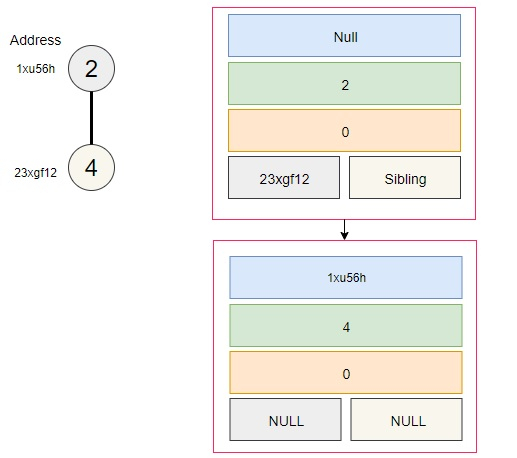# Memory representation of Binomial Heap in C++

## What is a Binomial Tree?

Binomial tree is an ordered tree data structure, let's say, B0 consists of a single node whereas a binomial tree represented as Bk consists of two binomial trees i.e. Bk-1 which are linked together. The root of one binomial tree is the leftmost child of the root of the other binomial tree. Binomial tree is mostly used for fundamental and technical analysing of assets or the stocks.

The nodes of a binomial tree represent the intrinsic value of an asset. It helps investors or buyers of the markets to analyze the right time and value for investment.

## What is a Binomial Heap?

Binomial heap is a data structure which is formed with the combinations of multiple binomial trees.

Properties of a binary heap H are-:

• Each binomial tree in H is heap-ordered. So the key of a node is greater than or equal to the key of its parent.

• There is at most one binomial tree in H, whose root has a given degree.

## Example of a binary heap is-: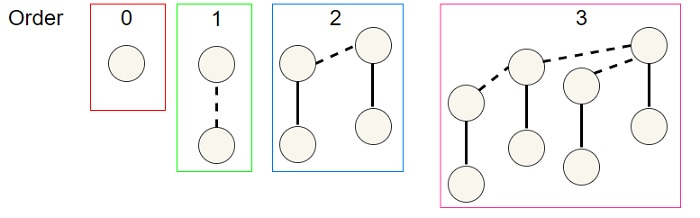## Memory representation of Binomial Heap node

Each node of a binary heap is representation in a memory with the 5 fields i.e.

• Parent Pointer -: It will store the address of a parent node such that it will be linked to other nodes in a binary heap structure.

• Key-: It will store the data or key which a node is holding.

• Degree-: it will specify the degree or the level of a binary heap node.

• Left child pointer-: It will store the address of an immediate left child to connect it with the left node if applicable.

• Sibling pointer-: it will store the address of the immediate sibling.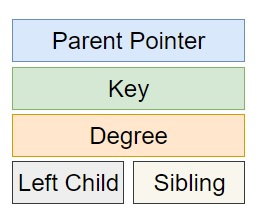## 1. Single node memory representation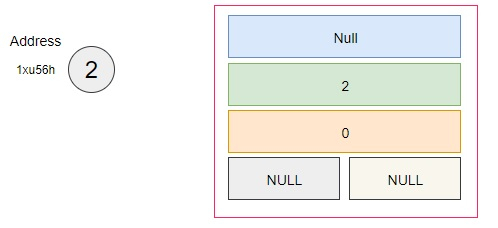## 2. Parent and child node memory representation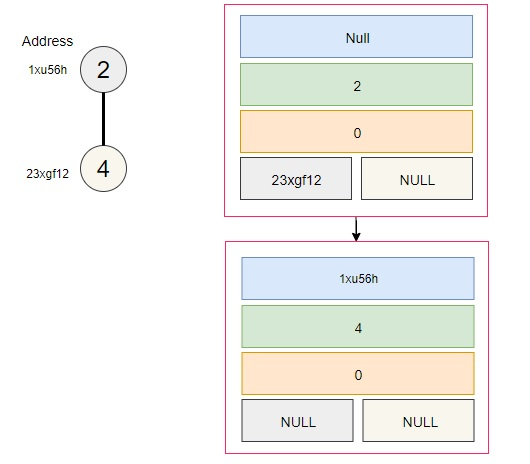## 3. sibling nodes memory representation